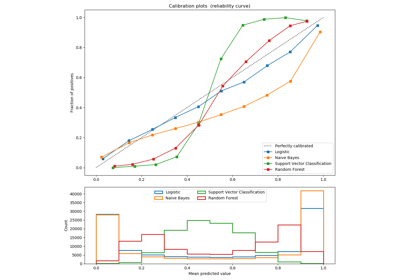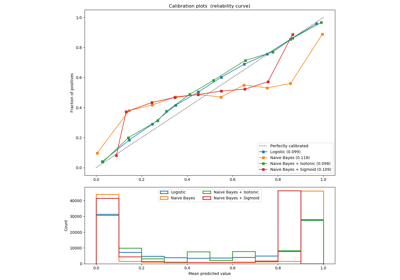/scikit-learn

# sklearn.calibration.calibration_curve

`sklearn.calibration.calibration_curve(y_true, y_prob, normalize=False, n_bins=5)` [source]

Compute true and predicted probabilities for a calibration curve.

The method assumes the inputs come from a binary classifier.

Calibration curves may also be referred to as reliability diagrams.

Read more in the User Guide.

Parameters: `y_true : array, shape (n_samples,)` True targets. `y_prob : array, shape (n_samples,)` Probabilities of the positive class. `normalize : bool, optional, default=False` Whether y_prob needs to be normalized into the bin [0, 1], i.e. is not a proper probability. If True, the smallest value in y_prob is mapped onto 0 and the largest one onto 1. `n_bins : int` Number of bins. A bigger number requires more data. `prob_true : array, shape (n_bins,)` The true probability in each bin (fraction of positives). `prob_pred : array, shape (n_bins,)` The mean predicted probability in each bin.

#### References

Alexandru Niculescu-Mizil and Rich Caruana (2005) Predicting Good Probabilities With Supervised Learning, in Proceedings of the 22nd International Conference on Machine Learning (ICML). See section 4 (Qualitative Analysis of Predictions).

## Examples using `sklearn.calibration.calibration_curve`Comparison of Calibration of ClassifiersProbability Calibration curves